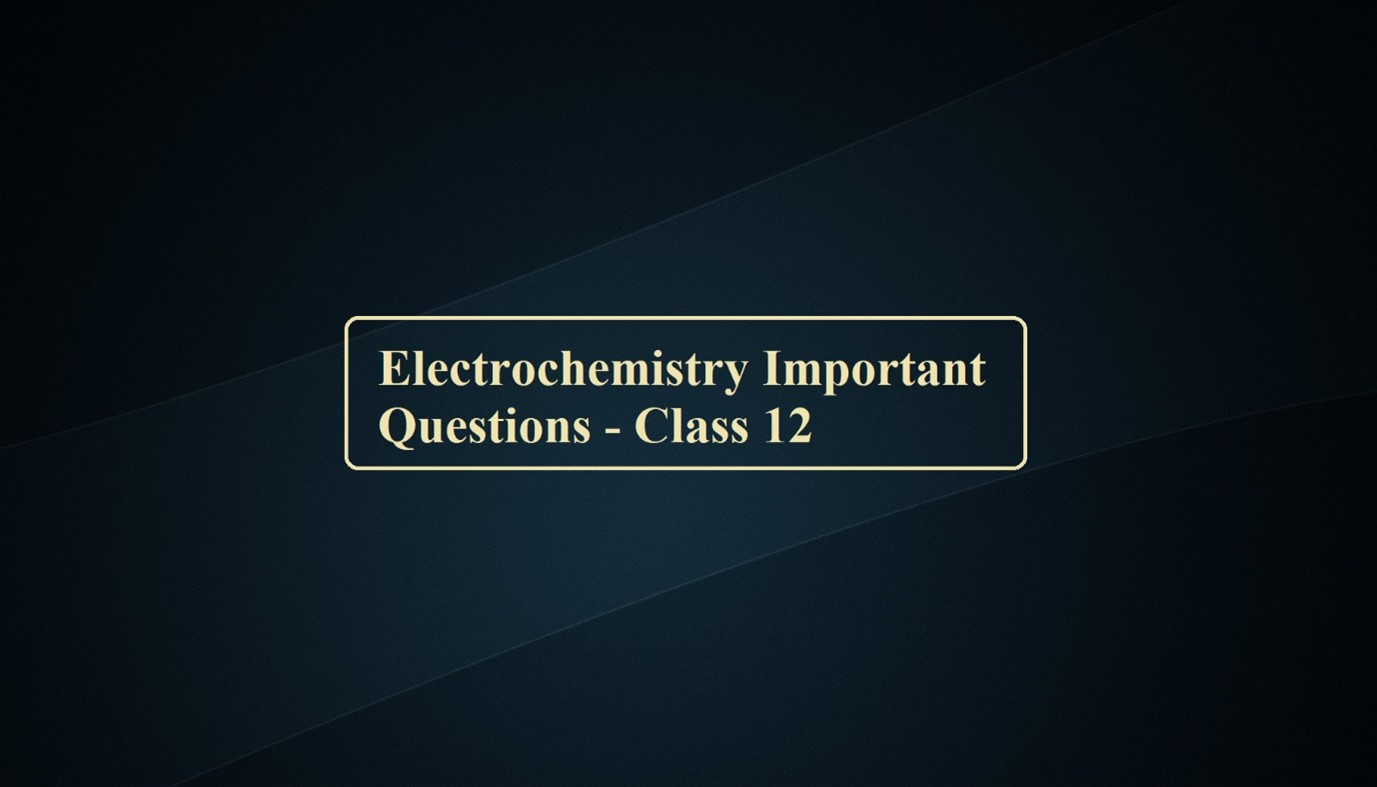# Electrochemistry Important Questions – Class 12# Electrochemistry Important Questions – Class 12

Electrochemistry Important Questions

Que 1. Why does a cell stop working after some time?

Que 2. What is the electrolyte used in a dry cell?

Que 3. Why can a lead storage cell be recharged?

Que 4. Why does a dry cell become dead after a long time even if it has not been used?

Que 5. Mention the reaction occurring at (i) anode and (ii) cathode during the working of mercury cell.

Que 6. Name any two metals which can be used for the cathodic protection of iron?

Que 7. Why is it that aluminium metal cannot be obtained by electrolysis of an aqueous solution of a salt of aluminium?

Que 8. Why does an alkaline medium inhibit the rusting of iron?

Que 9. Which product is formed at the anode during electrolysis of fused calcium hydride?

Que 10. What is the use of platinum foil in SHE?

Que 11. Rusting of iron is quicker in saline water than in ordinary water. Why?

Que 12. Explain the term electrolysis. Write the reactions at cathode and anode when molten NaCl is electrolysed.

Que 13. What are electrochemical equivalent and overvoltage?

Que 14. State two advantages of H2 – O2 fuel cell over ordinary cells?

Que 15. Define conductivity and molar conductivity for the solution of an electrolyte?

Que 16. The chemistry of corrosion of iron is essentially an electrochemical phenomenon. Explain the reactions occurring during the corrosion of iron.

Que 17. What is the difference between metallic conductors and electrolytic conductors?

Que 18. Define Kohlrausch law. How can it be used to find the degree of dissociation of a weak electrolyte?

Que 19. Calculate the potential of the hydrogen electrode in contact with a solution whose pH is 10.

Que 20. What is meant by standard emf of the cell? Derive the expression between standard emf of the cell and equilibrium constant.

Que 21. What advantages do fuel cells have over conventional methods of generating electrical energy?

Que 22. State relationship among cell constant of a cell resistance of the solution in a cell and conductivity of the solution. How is the molar conductivity of a solute is related to the conductivity of its solutions?

Que 23. Crude copper-containing iron and silver as contaminations are subjected to an electrorefining process. Answer the following with respect to the process.
(i) Name the material of the anode electrode, a cathode electrode and electrolyte.
(ii) Which electrode will lose or gain weight and why?
(iii) Why the loss and gain in the mass of electrodes be same or different.
(iv) Where will contamination go?

Que 24. (i) What is the relationship between Gibb’s free energy of the cell reaction in a galvanic cell and the emf of the cell? When will the maximum work be obtained from a galvanic cell?
(ii) Write the application of the electrochemical series.

Que 25. (a) Give reasons for the following:
(i) Rusting of iron is quicker in saline water than in ordinary water.
(ii) Aluminium metal cannot be produced by the electrolysis of aluminium salt.
(b) Resistance of a conductivity cell filled with 0.1 M KCl solution is 100 ohms. If the resistance of the same cell when filled with 0.02 M KCl solution is 520 ohms, calculate the conductivity and molar conductivity of 0.02 M KCl solution. The conductivity of 0.1 M KCl solution is 1.29 S m-1.

Electrochemistry Important Questions

Answers Electrochemistry Important Questions – Class 12

Class 12 Chapter Electrochemistry Test Paper – 1

Class 12 Electrochemistry Test Paper – 2